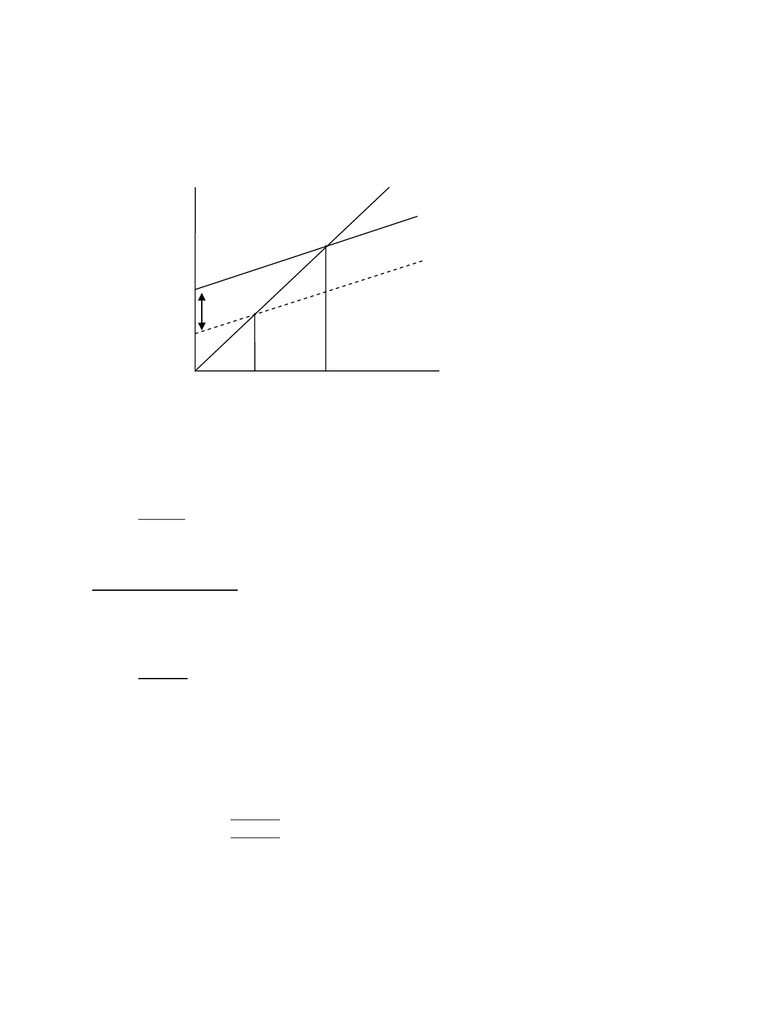# Lecture 30-The Multiplier

75 views5 pages
School
Department
Course
ProfessorThursday, February 4th, 2009.
The Multiplier
Autonomous Expenditure Ù Independent of charges in Y [GDP]
Induced Expenditure Ù Varies as Y [GDP] varies
Change in Autonomous Expenditure => shift in AE Schedule => new intercept of AE
Schedule
The Multiplier Concept
1. ¨< PXOWLSOLHU¨ Autonomous Expenditure
Example: ¨ \$XWRQRPRXV([SHQGLWXUH¨,
2. Induced consumption expenditure is key
Round #1 ¨I = 10 !¨< 
Round #2 ¨&PSF¨<   !¨Y = 9
Round #3 ¨&PSF¨<  !¨< 8.1
** mpc = marginal propensity to consume
Round #1 ¨ Autonomous Expenditure
Round #2 Induced Consumption Expenditure
Round #3 Induced Consumption Expenditure
** expenditure = income
=/[A[
450
C + I = AE
Y National Output
Aggregate
Planned
Expenditure
45°
¨I = 10
350
www.notesolution.com
Unlock document

This preview shows pages 1-2 of the document.
Unlock all 5 pages and 3 million more documents.

Already have an account? Log inThe Multiplier: Value
1. Slope of AE schedule
AE = C + I = 10 + 0.9Y + 25 = 35 + 0.9Y
Slope = 0.9
2. Multiplier = 1 .
1 ± slope of AE schedule
= 1 .
1 ± 0.9
= 10
Observation: In this model, slope of AE Schedule is mpc, so multiplier is 1___
1 ± mpc
Extended Model
AE = C + I + G + X ± M
Government Sector
G = Government expenditure
T = Taxes
(Note: T affects AE indirectly by influencing C)
Foreign Sector
X = Exports
M = Imports
Government Spending and Taxes
1. Spending __
G = G political determinants
2. Taxes
2.1 T = t * Y where t = marginal tax rate
T = 0.2 Y numerical example
2.2 YD (Disposable Income) = Y ± T
C = C0 (constant) + C1 (mpc) YD
= C0 + C1 (Y ± T)
Consumption and Real GDP (National Income)
C = 10 + 0.9 YD Note: YD
T = 0.2 Y
www.notesolution.com
Unlock document

This preview shows pages 1-2 of the document.
Unlock all 5 pages and 3 million more documents.

Already have an account? Log in

# Get OneClass Notes+

Unlimited access to class notes and textbook notes.

75% OFF
\$8 USD/m
###### Monthly
\$30 USD/m
You will be charged \$96 USD upfront and auto renewed at the end of each cycle. You may cancel anytime under Payment Settings. For more information, see our Terms and Privacy.
Payments are encrypted using 256-bit SSL. Powered by Stripe.